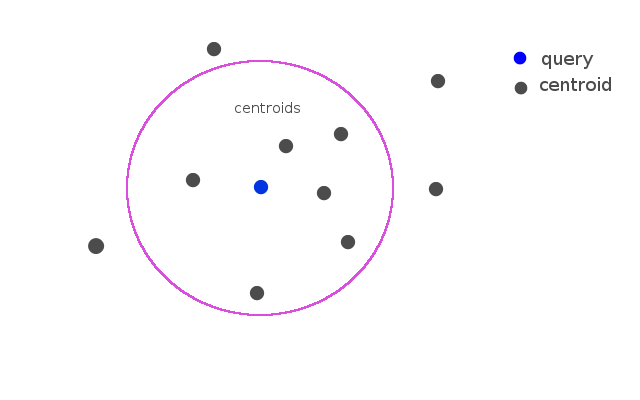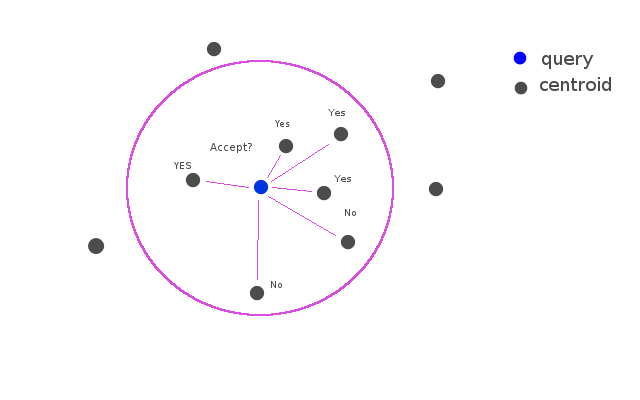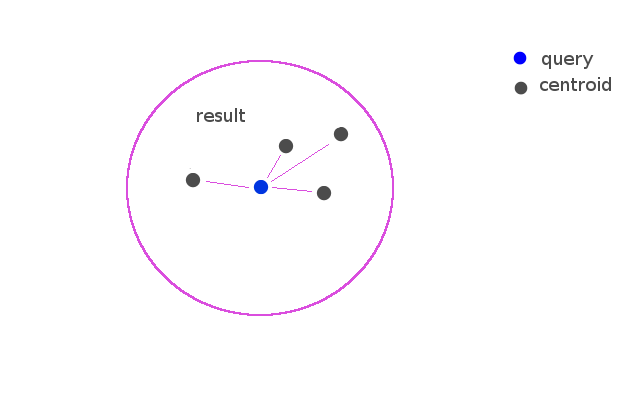{{ message }}

Code for Large Scale Hierarchical Text Classification competition. Final place: 3rd

Switch branches/tags
Nothing to show

## Files

Failed to load latest commit information.

Code for Large Scale Hierarchical Text Classification competition.

http://www.kaggle.com/c/lshtc

# Summary

a centroid-based flat classifier.

## Prediction

1. Selecting k-class from near the query with nearest centroid classifier.
2. Judging with binary classifier whether the query can be accepted to class.

(predict.cpp)Selecting k-candidate classes that centroid of class close to the query.Selecting classes that binary classifier of class returns p > 0.5. (Implementation of the binary classifier is logistic regression)## Training

For each data points..

1. Selecting k-class from near the data point with nearest centroid classifier.
2. Adding the data point as training data to dataset for each classes.

(prefetch.cpp)

For each classes..

1. Learning the binary classifier using own dataset.

(train.cpp)

## What are the feature

using variant TF-IDF.

``````tf = log(number_of_term_occurs_in_document + 1)
idf = log(total_number_of_documents / (number_of_documents_containing_term + 1)) + 5
tfidf = tf * idf
``````

and feature vector is normalized by L2 norm. (code: tfidf_transformer.hpp)

## What are the metric for Centroid Classifier

using cosine similarity.

• Ubuntu 13.10
• g++ 4.8.1
• make
• 32GB RAM

# How to Generate the Solution

``````make
./prefetch
./train
./predict
``````

NOTE: ./prefetch is very slow. probably processing time exceeds 15 hours.

# MISC programs

## Running the Validation Test

``````./vt_prefech
./vt_train
./validation
``````

## Simple k-NN baseline

running the validation test.

``````./vt_knn
``````

generating the sumission.txt.

``````./knn
``````

## Simple Nearest Centroid Classifier

running the validation test.

``````./vt_ncc
``````

generating the sumission.txt.

``````./ncc
``````

## Figure

ModelLBMaFTraining TimePrediction Time
k-NN0.23088n/a10 minutes
NCC0.2893180 seconds2 hours
NCC+BC0.3302515 hours2 hours

Code for Large Scale Hierarchical Text Classification competition. Final place: 3rd